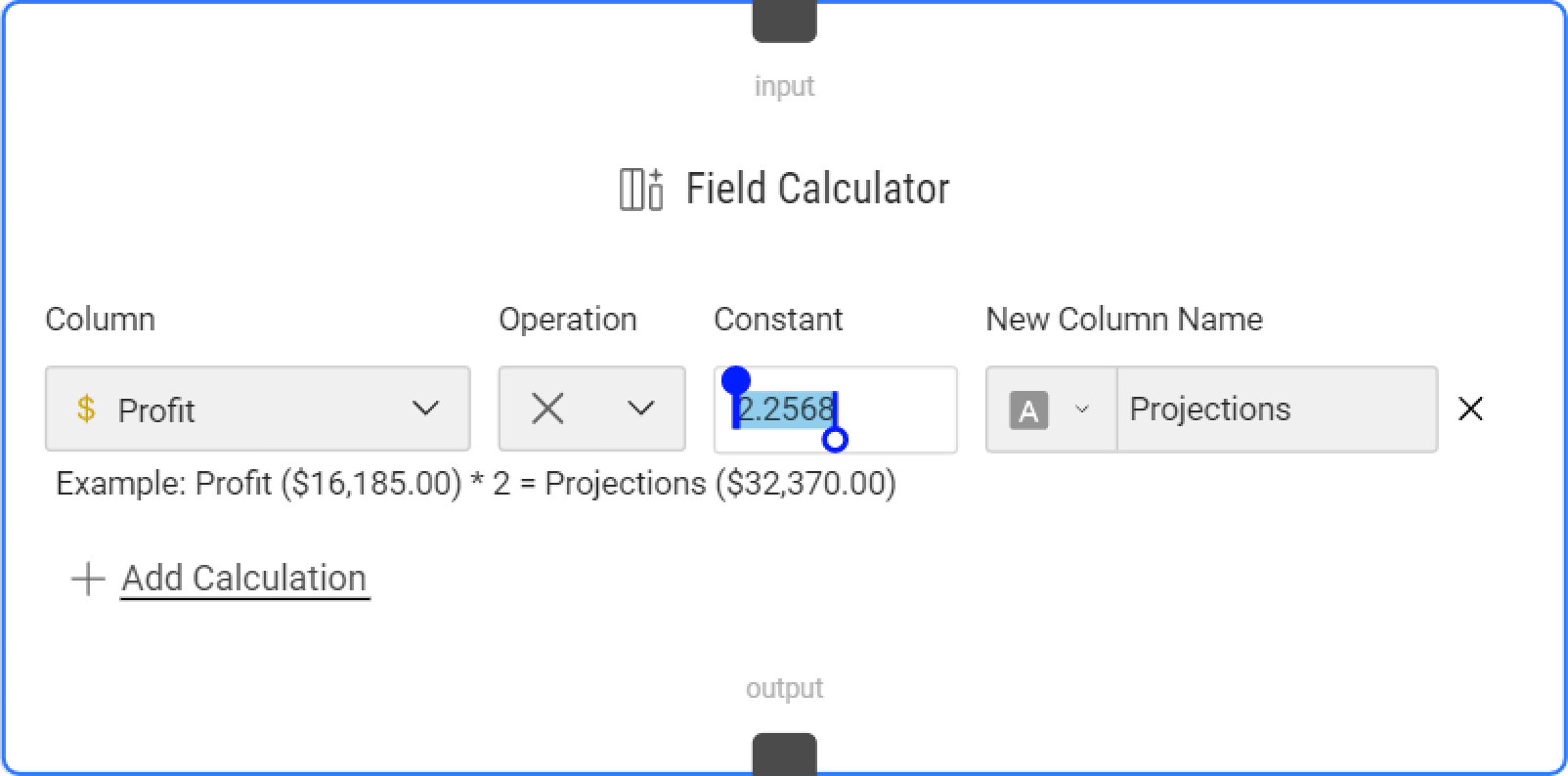# Transform Columns by a Constant ValueTransform values in a column by a constant value. You can pick any column and apply math operations to the values using a number.

## How Do I Add The Field Calculator Node?

Add a Field Calculator to any node in your Dataflow. Select any node and use the Transform (+) button to open the list of options to select Field Calculator. Note that you could work with the node in the Dataflow tab or use the Properties panel.

## What Are The Basics?

Creating a new field calculation is as simple as the following steps:

1. Select a column in your data.
2. Select an operation and enter constant.
3. Select a data type.
4. Give a name to the new column.

### 1. Select a column in your data.

Use the left-most dropdown to pick from the list of columns (data fields). You could click one or many or select all to choose all fields.

### 2. Select an operation and enter a constant.

Use the second dropdown to choose a math operation. The following mathematical operations are possible:

2. subtract (-) subtract all values by a constant value.
3. multiply (*) multiply all values by a constant value.
4. divide (/) divide all values by a constant value.
5. pow() raise all values in a column to the power of n (constant value).

### 3. Select a data type.

Use the dropdown within the text box to select a data type for the new column. By default, the node will auto-detect the data type in the field calculation and assign the same type in the new column.

### 4. Give a name to the new column.

Use the text box to enter a new name for the resulting column.

## How Do I Add, Re-Order, and Delete Calculations?

The Add Calculation button allows you to add as many calculations as you wish. You can use the drag bar on the left to re-order calculation upwards or downwards to the desired position.

Each field calculation you create will add a new column to the right of your data. You could also delete a field calculation by clicking on the "x" button to the right of the New Column Name.